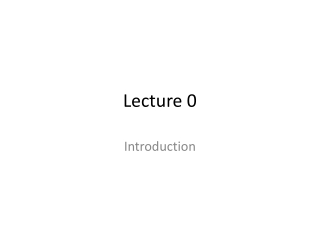DownloadDownload PresentationLecture 0

# Lecture 0

Download Presentation## Lecture 0

- - - - - - - - - - - - - - - - - - - - - - - - - - - E N D - - - - - - - - - - - - - - - - - - - - - - - - - - -
##### Presentation Transcript

1. Lecture 0 Introduction

2. Course Information • Your instructor: • Hyunseung (pronounced Hun-Sung) • Or HK (not Hong Kong ) • E-mail: khyuns@wharton.upenn.edu • Lecture: • Time: Mon/Tues/Wed/Thurat 10:45AM-12:15PM • Location: F45 JMHH • Office Hours: • 434 JMHH • Mon/Tues/Wed/Thur: 12:15PM-1:30PM (after lec.) • Course website: stat.wharton.upenn.edu/~khyuns/stat431/

3. Textbook: • None required • Recommended textbooks are on reserve at Van Pelt (2nd floor) • R • Free and widely used software (in academia) for data analysis • Download it from www.r-project.org • Grading: • 25% assignments, 35% weekly quizzes, and 40% final project • Assignments handed out every Monday. Due following Monday BEFORE CLASS!!! Collaboration highly encouraged, but final write-up must be prepared individually • Weekly quizzes given every Mondayat the beginning of class. Quizzes based on the assignment and the prior week’s lectures

4. Final Project • Goal • Analyze a real-world data set of your choosing • Provide numerical and theoretical justification of your analysis • Details • May work in groups up to three people • Turn in (1) a one-page executive summary of your analysis and (2) a technical report containing all your analysis

5. Prerequisite • “Fluency” with basic probability and analysis • Random variables • Probability distributions, Joint distributions, conditional probability • Independence/Correlation/Covariance • Law of Large Numbers • Central Limit Theorem • Moment generating functions • Multivariable calculus is required. • Linear algebra and R are not required, but useful

6. Statistical Inference in a Nutshell

7. Topics Covered • Gather Data: Population/Sample, Sampling Procedures • Summarize Data: • Mean, variance, risk, bias-variance trade-off • Histograms, Quantile-Quantile Plots, Scatterplots • Inference: Sampling distributions (e.g Chi-square, t, and F distributions) • Inference: One-Sample and Two-Sample Hypothesis Testing • Derivation of confidence intervals • Type I and II Error, Statistical power • Factorial Design (Chi-square Test for Independence) • Inference: Regression • Simple linear regression • Multiple linear regression: ANOVA, MANOVA, ANCOVA, polynomial regression, weighted least squares regression • Generalized linear models (GLMs): Logistic regression, logit regression, Poisson regression, probit regression • Time Series Models: AR and ARMA models • Model Diagnostics: Stepwise, Lasso, Ridge, AIC/BIC/Mallow’s Cp

8. Additional Topics (if we have time) • Nonparametric Regression: • Moving average estimators • Kernel methods • B-splines • Nonparametric Inference • Permutation Test • Welch’s Test, Signed-Rank Test, Kolmogorov-Smirnov Test • Bootstrap, Bayesian Inference, and Computation-based Inference • Multivariate Methods: • PCA and CCA • SVD • Likelihood-based Inference • Maximum Likelihood Estimators (MLE), Inference on MLEs

9. Questions?